150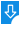10
2585

# Schr?dinger Equation Eigensystem Model  Free Download

Schr?dinger Equation Eigensystem Model Description:
Schr?dinger Equation Eigensystem Model is a small simulation that tries to solve the time-independent Schr?dinger Equation with arbitrary potential energy V(x) using the Numerov method recast on a discrete lattice. An user can adjust the location of hard walls, as well as the potential in the region between the walls. Schr?dinger Equation Eigensystem Model is developed in the Java programming language and can be run on Mac OS X, Windows and Linux.Schr?dinger Equation Eigensystem Model: Schr?dinger Equation Eigensystem Model for Mac, Simulation that solves the Schr?dinger Equation
Publisher: Wolfgang ChristianDownload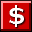Buy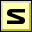Screenshot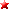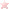Rate it
Category: Math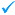Publisher: Wolfgang Christian    More titles >>
Last Updated: 01/06/2019
Requirements: Mac OS X